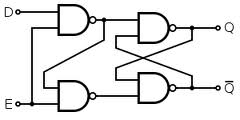## D-type Flip Flops Assignment Help

Assignment Help: >> Flip-Flops - D-type Flip Flops

D-type Flip Flops:

If a JK Flip Flop is changed by adding an inverter at the input as illustrated in Figure (a) thereby J and K inputs are always complementary to each other then we call such type of Flip-Flops as a D-type Flip Flops. Such Flip Flops behaves as a delay unit where the output follows the input which is delayed by a bit time, i.e. Qn + 1 at the (n + 1)th time instant is equal to Dn at the nth time instant. This can be verified from the truth table of JK Flip Flop when Dn = Jn = K¯n = 1, then Qn + 1 = 1, when Dn = Jn = Kn = 0, then Qn + 1 = 0. Therefore the truth table for D Flip Flop can be summed up to this Boolean equation: Qn + 1 = Dn. That is the output Qn + 1 after n + 1 bit time is equal to the input Dn at the previous n bit time instant. We may also realize a D-type Flip Flop from SR Flip Flop by making the same connection in the inputs S and R as illustrated in Figure (b). Both of the JK type and SR type Flip Flop has the same truth table as depicted in Figure (c).(a)                                      (b)                         (c)

Figure: (a) JK Type, (b) SR Type D Flip Flop